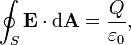Home » Posts tagged 'gauss theorem'

# Tag Archives: gauss theorem

## Gauss Theorem – Electrostatics

“WHAT IS THE  MAGNITUDE OF ELECTRIC  FIELD IN THE  GAUSSIAN SURFACE OF  A CUBE,AT ITS FACE ,AT ITS CENTRE, AT ITS CORNERS OR AT ANY OTHER POINT INSIDE THE CUBE. ALSO TELL ME THE THE WAY TO KNOW IT.”

Ans:

According to Gauss Theorem, the total electric flux through a closed surface,The Gaussian surface is an imaginary surface. So, for calculating the electric field at a point using Gauss theorem, we have to imagine a Gaussian surface symmetric with the given charge distribution.

I have assumed that there is a point charge Q at the centre of the cube.

At its face (at a point on the face on the line radially outwards from the point charge at the centre of the cube and perpendicular to the face)

The distance is equal to half the length of side of the cube (Say L).

On the corner,

Calculate the distance from the centre of the cube to its corner and replace (L/2) in the above equation with that distance.

## A Question from Electric Flux and Gauss Theorem

How can you prove that dS in φ =E.dS is dS Cos θ if the area is tilted at an angle ? I need the mathematical Steps.

Ans;

Dear Zeenath,
Electric Flux is defined as the total no of field lines passing normal to the surface. So while calculating, we need to consider the area of the surface normal to the field lines only. That is why we take the dot product of E and ds, where ds is the area vector (not just the area: Area vector is a vector whose magnitude is equal to the area and dirtected normal to the surface.)
Then by definition of dot product,
dφ =E.dS = EdS cos θ
which gives the component of E and the component of area vector in the direction of E (When area vector is in the direction of E, the actual component of area is perpendicular to E)
Hope the matter is clear.
Mathew Abraham

### Hits so far @ AskPhysics

• 2,214,936 hits

### CBSE Exam Count Down

AISSCE / AISSE 2020February 26, 2020
Board Exams for the core subjects of CBSE may start from 26 February (Class X) and 27 February (Class XII)